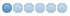• dagrende
• Offline
• New Member
•20 Sep 2009 19:52 #847 by dagrende
I have successfully run stepconf. When I start emc2 axis with the saved config, the homing menus are disabled.
I assume that the homing parameters for the axes are wrong and have tested different value combinations without success.

What are the rules that must be met for the homing function to be enabled?

Here are the axis part of my ini file (i also attach the whole ini file):
[AXIS_0]
TYPE = LINEAR
HOME = 0.0
MAX_VELOCITY = 20.0
MAX_ACCELERATION = 50.0
STEPGEN_MAXACCEL = 62.5
SCALE = 160.0
FERROR = 1
MIN_FERROR = .25
MIN_LIMIT = -250.0
MAX_LIMIT = 250.0
HOME_OFFSET = -245.000000
HOME_SEARCH_VEL = 0.050000
HOME_LATCH_VEL = 0.050000
HOME_SEQUENCE = 1

[AXIS_1]
TYPE = LINEAR
HOME = 0.0
MAX_VELOCITY = 15.0
MAX_ACCELERATION = 25.0
STEPGEN_MAXACCEL = 31.25
SCALE = 160.0
FERROR = 1
MIN_FERROR = .25
MIN_LIMIT = -125.0
MAX_LIMIT = 125.0
HOME_OFFSET = -120.000000
HOME_SEARCH_VEL = 0.050000
HOME_LATCH_VEL = 0.050000
HOME_SEQUENCE = 2

[AXIS_2]
TYPE = LINEAR
HOME = 120.0
MAX_VELOCITY = 4.0
MAX_ACCELERATION = 5.0
STEPGEN_MAXACCEL = 6.25
SCALE = 1600.0
FERROR = 1
MIN_FERROR = .25
MIN_LIMIT = 119.999
MAX_LIMIT = 120.001
HOME_OFFSET = -5.000000
HOME_SEARCH_VEL = -0.050000
HOME_LATCH_VEL = -0.050000
HOME_SEQUENCE = 0

Regards,
Dag Rende

21 Sep 2009 11:56 #852 by BigJohnT
Replied by BigJohnT on topic Re:homing menus disabled
You have to toggle the E-Stop off (the red circle with the X) and turn on the Machine Power (the red square with the I).

John

•